﻿ Factorial Analysis of Lead Bioextracted Using Mixed Cultures of Acidithiobacillus Ferrooxidans and Acidithiobacillus Thiooxidans on Galena

### Factorial Analysis of Lead Bioextracted Using Mixed Cultures of Acidithiobacillus Ferrooxidans and A...

C. I. Nwoye, E. M. Ameh, L. C. Oshionwu, M. A. Allen, M. O. Nwankwo, N. E. IdenyiOPEN ACCESSPEER-REVIEWED

## Factorial Analysis of Lead Bioextracted Using Mixed Cultures of Acidithiobacillus Ferrooxidans and Acidithiobacillus Thiooxidans on Galena

C. I. Nwoye1,, E. M. Ameh2, L. C. Oshionwu3, M. A. Allen4, M. O. Nwankwo3, N. E. Idenyi3

1Department of Metallurgical and Materials Engineering, Nnamdi Azikiwe University, Awka, Nigeria

2Department of Metallurgical and Materials Engineering, Enugu State University of Science & Technology, Enugu, Nigeria

3Department of Industrial Physics, Ebonyi State University, Abakiliki, Nigeria

4Department of Mechanical Engineering, Micheal Okpara University, Umuahia, Nigeria

### Abstract

This paper presents lead bioextraction using mixed cultures of Acidithiobacillus Ferrooxidans (ATF) and Acidithiobacillus Thiooxidans (ATT) on galena. The effect of leaching time at constant leaching temperature and ore mass-input were investigated. X-ray diffractometry (XRD) analysis of the galena used was carried out to evaluate the phases in the ore. Scanning electron microscopy (SEM) was carried out on reaction residues generated at different leaching times. The XRD analysis of the ore shows that it was composed of phases such as PbS, ZnS, ZnO and Cu64O with PbS dominating. The results of the experiment indicate that the concentrations of extracted lead increases with increase in the leaching time which ranged from 70 - 420 hrs. SEM of reaction residues show in some cases slight slimy substances suspected to be secretion from the microbes. A multivariate empirical model was derived, validated and used for the factorial analysis of extracted lead. The validity of the derived model expressed as; ξ - 0.258 = 0.0162 ɤ + 0.0002ϑ - 0.001₰ was rooted on the core model expression ξ - 0.258 = 0.0162 ɤ + 0.0002ϑ - 0.001₰ where both sides of the expression are correspondingly approximately equal. Regression model was used to generate results, and its trend of distribution was compared with that from experiment and derived model as means of verifying its validity. The results associating this verification translated into very close alignment of curves and significantly similar trend of data point’s distribution for experimental (ExD), derived model (MoD) and regression model-predicted (ReG) results. Evaluations from generated results indicated that extracted lead concentration per unit leaching time and final solution pH as obtained from experiment, derived model & regression model were 0.0171, 0.0162 & 0.0190 g/dm3 hr-1 and 6.386, 6.0467 & 7.1083 respectively. The standard errors in predicting the extracted lead concentrations for each values of the leaching times and final solution pHs as obtained from experiments, derived model & regression model-predicted results were 0.3666, 0.0003 & 6.901 x 10-5 and 1.7626, 1.3135 & 1.5437 respectively. The model operates with a confidence and reliability level range: 91-99.86 % at recorded deviation range: 0.14 - 8.51 (of the model-predicted extracted lead concentration from experimental results).

### At a glance: Figures

12345
Prev Next

• Nwoye, C. I., et al. "Factorial Analysis of Lead Bioextracted Using Mixed Cultures of Acidithiobacillus Ferrooxidans and Acidithiobacillus Thiooxidans on Galena." Biomedicine and Biotechnology 2.2 (2014): 29-36.
• Nwoye, C. I. , Ameh, E. M. , Oshionwu, L. C. , Allen, M. A. , Nwankwo, M. O. , & Idenyi, N. E. (2014). Factorial Analysis of Lead Bioextracted Using Mixed Cultures of Acidithiobacillus Ferrooxidans and Acidithiobacillus Thiooxidans on Galena. Biomedicine and Biotechnology, 2(2), 29-36.
• Nwoye, C. I., E. M. Ameh, L. C. Oshionwu, M. A. Allen, M. O. Nwankwo, and N. E. Idenyi. "Factorial Analysis of Lead Bioextracted Using Mixed Cultures of Acidithiobacillus Ferrooxidans and Acidithiobacillus Thiooxidans on Galena." Biomedicine and Biotechnology 2, no. 2 (2014): 29-36.

 Import into BibTeX Import into EndNote Import into RefMan Import into RefWorks

### 1. Introduction

The unavoidable need for intensive and extensive research and development aimed at enhancing methods of extracting lead from its natural ores has been significantly prompted by the wide spectrum of lead applicability. The conventional step-wise roasting or hydrometallurgical process has been the basic method of lead extraction from galena. Conventional method of lead extraction has been environmental unfriendly due to series of liberated gases in the course of the process. Air pollution resulting from the roasting of lead ore has drawn lots of severe legislation, co-opted with stiff penalties by the government. Based on the foregoing, development of viable and sustainable alternative methods including hydrometallurgical routes which does not only eliminates atmospheric pollution due to production of SO2, but remains environment friendly has been in progress.

Acid and alkaline leaching has gained very wide recognition as a viable and sustainable metal extraction route. This route gained a significantly high level of popularity due to its environment friendliness and ease of operation.

Bioleaching has since been used in the past in mineral pretreatment of refractory sulfides, mainly in the gold, copper and uranium benefit. This technology has been proved to be cheaper, more efficient and environmentally friendly than roasting and high pressure moisture heating processes . Microorganisms considered important in commercial mineral biooxidation processes are: Acidithiobacillus Thiooxidans, Acidithiobacillus Ferrooxidans, and Acidithiobacillus Caldus, Leptospirillum Ferrooxidans, and Acidiphilium Acidophilum . The microorganisms, acidithiobacillus Ferrooxidans are able to oxidize ferrous ions and the reduced sulphur compounds  while acidithiobacillus Thiooxidans are able to oxide only reduced sulphur compound summarized by the global reaction [4, 5]. ATT an extremely acidophidic but not ferrous iron oxidizing of the Thiobacillus, is not able to solubilise heavy metal minerals in culture. Nevertheless ATT plays a role in metal leaching. The solubilising of sulphidic minerals by ATF is increased by cooperation with ATT as compared with the effect of ATF alone. It is assumed  that the cause of this enhancement is the oxidation of elemental sulphur, by ATT culminating in the formation of hydrogen sulphide as a result of the oxidation by ferric ion according the equation:

ATF in co-operation with ATT, disintegrate sulphidic ferrous iron containing minerals by oxidation and bring them to solution. Also when ATT is used together with ATF, the concentration of leached metal is far greater than that obtained when only ATF is used.

Open-system predictive assessment of lead extraction rate during biooxidation of galena by acidithiobacillus ferrooxidans (ATF) has been carried out  based on the leaching time and final pH of leaching solution using a derived model. The model;(1)

indicates an logarithmic relationship between lead extraction rate and combined input of time and pH. Results were predicted using regression model (standard model) and then plotted along side with results from the experiment and derived model to compare their respective spread and trend so as to establish the degree of validity of the derived model.

The standard errors incurred in predicting lead extraction rate for each value of the leaching time and final leaching solution pH considered, as obtained from derived model and experiments are 5.15 x 10-4 and 4.66 x 10-4 % as well as 1.26 x 10-3 and 1.54 x 10-3 % respectively.

The correlations between lead extraction rate and leaching time as obtained from derived model and experimental results were evaluated to be same (0.9959), and between lead extraction rate and final pH of leaching solution 0.9596 and 0.9749 respectively. The concentration of lead extracted within a leaching time interval 210-490 hrs as obtained from derived model and experiment are 3.136 and 3.108 g/dm3 respectively.

Deviational analysis indicates that the maximum deviation of the model-predicted lead extraction rate from the corresponding experimental value is less than 10%. It was also found that the validity of the model is rooted on the core expression 7.4074 γ = - 0.1304 (ln t + ln α) + 1 where both sides of the expression are correspondingly approximately equal.

Studies  were carried out to ascertain the predictability of maximum lead extraction based on optimized leaching time during biooxidation of galena by Acidithiobacillus Thiooxidans. Series of experiments were carried out and a model was derived from the results to predict lead extraction rates and optimize the leaching time. Results generated from the research indicate that the concentration of extracted lead increases with increase in the leaching time. Evaluation of the derived model: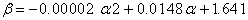(2)

(D-Model) to determine maximum lead extraction and the associated optimum leaching time gave 4.379 g/dm3 at 370 hrs respectively even though the model also predicted lead extraction: 4.371 g/dm3 at a leaching time of 350 hrs. This strongly implies that maximum lead extraction of ≈ 4.4 g/dm3 is achievable during the biooxidation process at a leaching time range 350-370 hrs.

Extracted lead concentrations per unit leaching time as obtained from experiment and derived model are 3.85 x 10-3 and 3.6 x 10-3 g/dm3 respectively. The validity of the model was rooted on the expression 0.6094 β = - 1.2188 x 10-5 α2 + 9.0189 x 10-3α + 1 where both sides of the expression are correspondingly approximately equal.

The maximum deviation of the derived model predicted extracted lead concentration from the corresponding experimental value was less than 8%.

The standard error (STEYX) in predicting the concentration of extracted lead for any value of leaching time used is 0.4017 compared to that of the experiment (0.4541).

The present work presents a factorial analysis of lead bioextraction (from galena) using mixed cultures of Acidithiobacillus Ferrooxidans and Acidithiobacillus Thiooxidans. An empirical model is expected to be formulated for this analysis.

### 2. Materials and Method

Galena from Ishiagu (Ebonyi state, Nigeria) was homogenized, crushed and sieved to a grain size of 100 μm using sieve analysis technique.

Figure 1. (a) galena (as mined) (b) crushed and ground galena (c) galena concentrate (d) galena concentrate sieved to 100 μm for the bioleaching process.

100 cm3 leaching medium (2.0 g/dm3 Fe (ii) sulphate liquid medium) supplemented with 6.0 g of 100 μm grain sized galena concentrate (loose particles) was put in 7 flasks. Bacteria: ATF and ATT were obtained from red soil shown in Figure 3 b. The volume of the bacteria (ATF + ATT) culture inoculum used was 12 cm3 for all the flasks. The 7th flask was without bacteria and is identified as control. The initial pH of each leaching medium used was 4.0. The experiments were started under exactly the same solution conditions of pH and redox potential (0.9926 V) of leaching medium in bacteria and uninoculated control leaching and spanned through 70 hrs. The leaching temperature was maintained at room temperature; 28C. The experiments were repeated for leaching times 140, 210, 280, 350 and 420 hrs and the corresponding leached out lead chemically analyzed. Detailed experimental procedures are stated in the report .

### 3. Results and Discussion

Variation of extracted lead with leaching time

The effect of leaching time at constant leaching temperature and ore mass-input indicates that the concentrations of extracted lead increases with increase in the leaching time which ranged from 70 - 420 hrs. Table 1 shows that for each experimental set up, the final solution pH drops compared to the initial solution pH. This was attributed to dissolution of hydrogen sulphide (H2S) in the leaching solution thereby enhancing the inherent acidity level.

#### Table 1. Variation of extracted lead concentration with leaching time and final solution pH

Formation of H2S was due to elemental sulphur oxidation by ATT as a result of oxidation by ferric ion. The action of ATT in the mixed culture of ATH + ATT was strongly believed to have significantly enhanced solubilising of PbS as a result of elemental sulphur oxidation. This is in line with previous research . Equations of these reactions are shown:(3)

This oxidation produces hydrogen ions which in turn attack the minerals according to the following equations: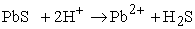(4)

The bioleaching process residues were very gray in colour (as shown in Figure 3a) irrespective of the process time used. The SEM analysis of the process residues at leaching times: 70 and 420 hrs shows whitish substance sparsely distributed on the lead structures (Figure 4 (a)-.(f)). This was suspected to be secretions from the bacteria used in the leaching process. The lead structures at 70 hrs process time (Figure 4 (a-c) show sleek-like and jelly-like appearance. This was attributed to the wriggling movement of the microbes in the course of their activities. In contrast, lead structures at 420 hrs process time (Figure 4 (d-f) were larger than those at 70 hrs process time. This was perceived as lead particles clogged together by large quantity of periodic sticky-secretions by the bacteria during the leaching process.

Figure 4. SEM of bioleaching residues (a), (b) and (c) after 70 hrs process time (d), (e) and (f) after 420 hrs process time
3.1. Model Formulation

Computational analysis of experimental results in Table 1; generated during the leaching process indicates that;(5)

Introducing the values of Se, S, K and N into equation (5) reduces it to;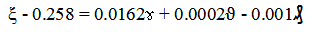(6)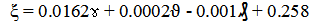(7)

Where

(ξ) = Conc. of extracted Pb (g/dm3)

(ɤ) = Leaching time (hr)

(ϑ) = Leaching temperature (C)

₰ = Mass-input of galena (g)

Se = 0.258, S = 0.0162, K = 0.0002 and N = 0.001

empirical constants determined using C-NIKBRAN 

### 4. Boundary and Initial Conditions

Consider galena placed in a flask containing leaching solution (2.0 g/dm3 Fe (II) liquid medium) and supplemented with 6.0 g of 100 μm grain sized galena concentrate. The flask atmosphere is not contaminated i.e. (free of unwanted bacteria, gases and dusts). Initially, atmospheric levels of oxygen are assumed just before the commencement of the process (due to air in the flask).

Range of leaching time: 70-420 hrs was used. Treatment temperature: 28C, initial pH: 4.0, redox potential: 0.9926, volume of leaching solution: 100 cm3 and ore grain size: 100 µm were also used. The boundary conditions are: flask oxygen atmosphere for enhancement of Fe (II) oxidation. At the bottom of the particles, a zero gradient for the gas scalar are assumed and also for the gas phase at the top of the particles. The sides of the particles are taken to be symmetries.

4.1. Model Validity

The validity of the model was strongly rooted in the core model equation (equation (6)) where both sides of the equation are correspondingly approximately equal.

#### Table 2. Variation of ξ - 0.258 with 0.0162 ɤ+ 0.0002ϑ - 0.001₰

Table 2 also agrees with equation (6) following the values of ξ - 0.258 and 0.0162ɤ + 0.0002ϑ - 0.001₰ evaluated from the experimental results in Table 1.

Furthermore, the derived model was validated by comparing the extracted lead concentrations as predicted by the model and obtained directly from the experiment. This was done using various evaluative techniques such as statistical, graphical, computational and deviational analysis.

4.2. Statistical Analysis
4.2.1. Standard Error (STEYX)

The standard errors in predicting the extracted lead concentrations for each value of the leaching times and final solution pHs as obtained from experiments & derived model-predicted results were 0.3666 & 0.0003 and 1.7626 & 1.3135 respectively.

Figure 5. Coefficient of determination between the extracted lead concentrations and leaching time as obtained from experiment
Figure 8. Coefficient of determination between the extracted lead concentrations and final solution pH as predicted by model
Figure 9. Comparison of extracted lead concentrations (relative to leaching time) as obtained from experiment and derived model
Figure 10. Comparison of extracted lead concentrations (relative to final solution pH) as obtained from experiment and derived model
Figure 11. Comparison of extracted lead concentrations (relative to leaching time) as obtained from experiment, derived model and regression model
Figure 12. Comparison of extracted lead concentrations (relative to final solution pH) as obtained from experiment, derived model and regression model

4.2.2. Correlation (CORREL)

The correlation coefficients between extracted lead concentration and leaching time as well as final solution pH were calculated from the results of derived model and experiment. This was done by considering the coefficients of determination R2 from Figure 5- Figure 8 and then evaluating them using the expression: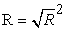(8)

The evaluated correlations (using Microsoft Excel version 2003) are shown in Table 3 and Table 4. These evaluated results indicate that the derived model predictions are significantly reliable, considering the proximate agreement between actual experimental and model-predicted results.

#### Table 4. Comparison of the correlations evaluated from derived model predicted and ExD results based on final solution pH

4.3. Graphical Analysis

Comparative graphical analysis of Figure 9 and Figure 10 shows extremely close alignment of the curves from the experimental (ExD) and model-predicted (MoD) extracted lead concentration. Furthermore, the degree of alignment of these curves is indicative of the proximate agreement between both experimental and model-predicted extracted lead concentration.

4.4. Comparison of Derived Model with Standard Model

The validity of the derived model was further verified through application of the regression model (Reg) (Least Square Method using Excel version 2003) in predicting the trend of the experimental results. Comparative analysis of Figure 11 and Figure 12 show very close alignment of curves which depicted significantly similar trend of data point’s distribution for experimental (ExD), derived model (MoD) and regression model-predicted (ReG) results of extracted lead concentration. Moreover, the evaluated correlations between extracted lead concentration and leaching time & final solution pH for regression model-predicted results gave 1.0000 & 0.8327 respectively. These values are in proximate agreement with both experimental and derived model-predicted results. The standard errors incurred in predicting the extracted lead concentration for each values of the leaching time and final solution, as evaluated from regression model-predicted results were 6.901 x 10-5 1.5437 % respectively.

4.5. Computational Analysis

Comparative analyses of the extracted lead concentrations evaluated from experimental, derived model-predicted and regression model predicted results were carried out to ascertain the degree of validity of the derived model. This was done by comparing evaluated results of extracted lead concentrations per unit leaching time and per unit final solution pH resulting from the leaching process.

4.5.1. Extracted Lead Concentration Per Unit Leaching Time

Pb/ ϑ (g/dm3 hr-1) was calculated from the equation;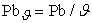(9)

Re-written as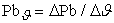(10)

Equation (10) is detailed as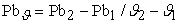(11)

Where

ΔPb = Change in the extracted lead concentration Pb 2, Pb 1 at leaching times ϑ 2 , ϑ 1.

Considering the points (70, 1.4101) & (350, 6.1996), (70, 1.392) & (350, 5.927) and (70, 1.1860) & (350, 6.5174) as shown in Figure 11, and designating them as (Pb1, ϑ1) & (Pb2, ϑ2) for experimental, derived model and regression model predicted results respectively, and then substituting them into equation (11), gives the slopes: 0.0171, 0.0162 and 0.0190 g/dm3 hr-1 respectively as their corresponding extracted lead concentration per unit leaching time.

4.5.2. Extracted Lead Concentration Per Unit Final Solution pH

Similarly, substituting into equation (11) points (2.63, 1.4101) & (3.38, 6.1996), (2.63, 1.392) & (3.38, 5.927) and (2.63, 1.1860) & (3.38, 6.5174) culled from Table 1 and Figure 12, as (Pb1, ξ1) & (Pb2, ξ2) for experimental, derived model and regression model predicted results respectively also gives the slopes: 6.386, 6.0467 and 7.1083 respectively as their corresponding extracted lead concentration per unit final solution pH. The proximity between values in each result set indicates significantly high validity level for the derived model.

4.6. Deviational Analysis

Critical Comparative analysis of extracted lead concentrations obtained from experiment and derived model show deviations on the part of the model-predicted values relative to values obtained from the experiment. This is attributed to the fact that the surface properties of the ore and the physiochemical interactions between the ore and bioleaching solution, which played vital roles during the extraction process, were not considered during the model formulation.

This invariably necessitated the introduction of correction factor, to bring the model-predicted extracted lead concentration to those of the corresponding experimental values.(12)

The deviation Dv, of model-predicted extracted lead concentration from the corresponding experimental result was given by

Figure 13. Variation of derived model predicted concentration of extracted lead with leaching time relative to its associated deviation from experimental results
Figure 14. Variation of deviation (of derived model predicted concentration of extracted lead from experimental result) with leaching time and final solution pH

Figure 13 and Figure 14 show that the maximum deviation of the model-predicted concentrations of extracted lead from the corresponding experimental values was less than 15% and quite within the acceptable deviation limit of experimental results. The model operates with a confidence and reliability level range: 91-99.86 %, at recorded deviation range: 0.14 - 8.51 (of the model-predicted extracted lead concentration from experimental results).

Consideration of equation (12) and critical analysis of Figure 5- Figure 8 as well as Figure 13 and Figure 14 show that the least and highest magnitudes of deviation of the model-predicted extracted lead concentration (from the corresponding experimental values) were - 0.14 and -14.93%. This invariably corresponds to extracted lead concentrations: 4.793 and 7.061 g/dm3 hr-1; leaching times: 280 and 420 hrs as well as final solution pHs: 3.22 and 3.9 respectively.

#### Table 5. Variation of correction factor (to model-predicted extracted lead concentration) with leaching time and final solution pH

Correction factor, Cf to the model-predicted results is given by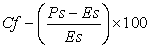(13)

Where

Es and Ps are extracted lead concentrations evaluated from experiment and derived model respectively.

Equations (12) and (13) indicate that correction factor is the negative of the deviation.

The correction factor took care of the negligence of operational contributions of surface properties of the ore and the physiochemical interactions between the ore and bioleaching solution, which actually played vital role during the leaching process. The model predicted results deviated from those of the experiment because these contributions were not considered during the model formulation. Introduction of the corresponding values of Cf from equation (13) into the model gives exactly the corresponding experimental values of extracted lead concentrations.

Equation (13) and critical analysis of Figure 11, Figure 12 and Table 4 indicate that the evaluated correction factors are negative of the deviation as shown in equations (12) and (13). Table 5, Figure 3- Figure 6 as well as Figure 11 and Figure 12 show that the least and highest correction factor (to the model-predicted extracted lead concentration) were + 0.14 and + 14.93% which corresponds to extracted lead concentrations: 4.793 and 7.061 g/dm3 mins-1; leaching times: 280 and 420 mins. as well as final solution pHs: 3.22 and 3.9 respectively.

It is important to state that the deviation of model predicted results from that of the experiment is just the magnitude of the value. The associated sign preceding the value signifies that the deviation is a deficit (negative sign) or surplus (positive sign).

### 5. Conclusion

Lead was extracted using mixed cultures of Acidithiobacillus Ferrooxidans (ATF) and Acidithiobacillus Thiooxidans (ATT) on galena. XRD analysis of the ore shows that it was composed of phases such as PbS, ZnS, ZnO and Cu64O with PbS dominating. The concentrations of extracted lead increased with increase in the leaching time which ranged from 70 - 420 hrs. SEM of reaction residues show in some cases slight slimy substances suspected to be secretion from the microbes. A multivariate empirical model derived, validated and used for the factorial analysis of bioextracted lead indicated that validity of the derived model was rooted on the core model expression ξ - 0.258 = 0.0162ɤ + 0.0002ϑ - 0.001₰ where both sides of the expression are correspondingly approximately equal. Evaluations from generated results indicated that extracted lead concentration per unit leaching time and final solution pH as obtained from experiment, derived model & regression model were 0.0171, 0.0162 & 0.0190 g/dm3 hr-1 and 6.386, 6.0467 & 7.1083 respectively. The standard errors in predicting the extracted lead concentrations for each values of the leaching times and final solution pHs as obtained from experiments, derived model & regression model-predicted results were 0.3666, 0.0003 & 6.901 x 10-5 and 1.7626, 1.3135 & 1.5437 respectively. The model operates with a confidence and reliability level range: 91-99.86 % at recorded deviation range: 0.14 - 8.51 (of the model-predicted extracted lead concentration from experimental results).

### References

  Makita, M., Esperon, M., Pereyra, B., Lopez, A., and Orrantia, E. (2004). Reduction of Arsenic Content in a Complex Galena Concentrate by Acidithiobacillus Ferrooxidans; Bio Med Central Biotechnology, 4: 22.In article  Rawlings, D. E. (2002). Heavy Metals Mining using Microbes Annual Review of Microbiology 2 (56): 65-91.In article CrossRef  Haver, F. P. and Wong, M. M. (1971). Recovering Elemental Sulfur from Non-Ferrous Minerals. Rep. Invest. U.S Bur. Mines 7474.In article  Sklodowska, R. (1990). Microbial Leaching of Blende Flotation Concentrate using Acidithiobacillus Ferrooxidans and Acidithiobacillus Thiooxidans; Physico Chemical Problems of Mineral Processing 37: 58.In article  C. I. Nwoye, J. U. Odo, S. O. Nwakpa and O. O. Onyemaobi (2013) Open-System Predictive Assessment of Lead Extraction Rate during Biooxidation of Galena by Acidithiobacillus Ferrooxidans. International Journal of Scientific & Engineering Research. 4 (9): 396-408.In article  C. I. Nwoye, F. Asuke, R. A. Ejimofor and O. O. Onyemaobi (2013). Biooxidation of Galena by Acidithiobacillus Thiooxidans (ATT) and Prediction of Maximum Lead Extraction Based on Optimized Leaching Time. International Science and Investigation Journal. 2 (5): 35-52.In article  Nwoye, C. I. (2008).Studies on the Bioleaching of Ishiagu Galena. Ph.D Thesis. Federal University of Technology, Owerri, Nigeria.In article  Nwoye, C. I. (2008). C-NIKBRAN: Data Analytical Memory-Software.In article